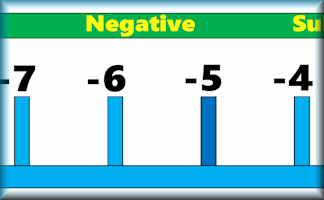Number LineLeftmost number: showing a

Digital DartsAn online darts game for one or two players requiring skill, strategy and mental arithmetic. So far this activity has been accessed 230082 times and 1529 Transum Trophies have been awarded for completing it.

Number Line

For the teaching of decimal fractions see the Decimals Line.

Change the numbers displayed on the number line by using the dropdown boxes above. The two arrows are available to drag up to the number line to show certain values.

This number line can help you explain directed number arithmetic. For example to show the calculation 6 - 10 you could start by moving the blue arrow to show positive six. Next use the red arrow to move ten units to the left (equivalent to minus ten) ending at minus four.

To show the calculation - 4 - - 7 begin with the blue arrow at minus four. The red arrow can then show that subtracting negative seven equates to a move to the right ending at positive three.

There are many more Negative Numbers activities on Transum.org. There are pupil activities, teacher resources and lesson starters.

If you are a Transum subscriber there is a colourful, printable Number Line banner to print out for your classroom. No Mathematics room should be without a number line on the wall for a quick and easy reference when adding and subtracting.Do you have any comments? It is always useful to receive feedback and helps make this free resource even more useful for those learning Mathematics anywhere in the world. Click here to enter your comments.For All: